Students can download 10th Science Chapter 2 Optics Questions and Answers, Notes, Samacheer Kalvi 10th Science Guide Pdf helps you to revise the complete Tamilnadu State Board New Syllabus, helps students complete homework assignments and to score high marks in board exams.

## Tamilnadu Samacheer Kalvi 10th Science Solutions Chapter 2 Optics

### Samacheer Kalvi 10th Science Optics Text Book Back Questions and Answers

Question 1.
The refractive index of four substances A, B, C and D are 1.31,1.43,1.33, 2.4 respectively. The speed of light is maximum in:
(a) A
(b) B
(c) C
(d) D
(a) A

Question 2.
Where should an object be placed so that a real and inverted image of same size is obtained by a convex lens:
(a) f
(b) 2f
(c) infinity
(d) between f and 2f
(b) 2f

Question 3.
Where should an object be placed so that a real and inverted image of the same size is obtained by a convex lens ______.
(a) f
(b) 2f
(c) infinity
(d) between f and 2f.
(b) 2fQuestion 4.
Magnification of a convex lens is _____.
(a) positive
(b) negative
(c) either positive or negative
(d) zero.
(b) negative

Question 5.
A convex lens forms a real, diminished point sized image at focus. Then the position of the object is at:
(a) focus
(b) infinity
(c) at 2f
(d) between f and 2f
(b) infinity

Question 6.
Power of a lens is -4D, then its focal length is:
(a) 4 m
(b) -40 m
(c) -0.25 m
(d) -2.5 m
(d) -2.5 m

Question 7.
In a myopic eye, the image of the object is formed _____.
(a) behind the retina
(b) on the retina
(c) in front of the retina
(d) on the blind spot.
(c) in front of the retina

Question 8.
The eye defect ‘presbyopia’ can be corrected by:
(a) convex lens
(b) concave lens
(c) convex mirror
(d) Bi focal lenses
(d) Bi focal lenses

Question 9.
Which of the following lens would you prefer to use while reading small letters found in a dictionary?
(a) A convex lens of focal length 5 cm
(b) A concave lens of focal length 5 cm
(c) A convex lens of focal length 10 cm
(d) A concave lens of focal length 10 cm
(d) A concave lens of focal length 10 cm

Question 10.
If VB, VG, VR be the velocity of blue, green and red light respectively in a glass prism, then which of the following statement gives the correct relation?
(a) VB = VG = VR
(b) VB > VG > VR
(c) VB < VG < VR
(d) VB < VG > VR
(c) VB < VG < VRII. Fill in the blanks.

1. The path of the light is called as ………
2. The refractive index of a transparent medium is always greater than ……….
3. If the energy of incident beam and the scattered beam are same, then the ………. scattering of light is called as scattering ……….
4. According to Rayleigh’s scattering law, the amount of scattering of light is inversely proportional to the fourth power of its ……….
5. Amount of light entering into the eye is controlled by ……….

1. ray
2. unity
3. elastic
4. wavelength
5. iris

III. True or False. If false correct it.

1. Velocity of light is greater in denser medium than in rarer medium.
2. The power of lens depends on the focal length of the lens.
3. Increase in the converging power of eye lens cause ‘hypermetropia’
4. The convex lens always gives small virtual image.

1. False – Velocity of light is greater in rarer medium than in denser medium.
2. True
3. True
4. False – The convex lens does not give small virtual image always.

IV. Match the following.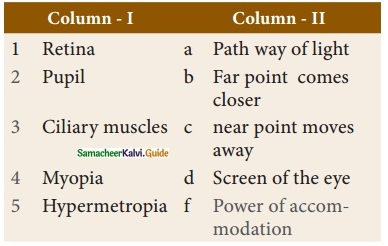1. d
2. a
3. e
4. b
5. cV. Assertion and reasoning type.

Mark the correct choice as-
(a) If both assertion and reason are true and reason is the correct explanation of assertion.
(b) If both assertion and reason are true but reason is not the correct explanation of assertion.
(c) Assertion is true but reason is false.
(d) Assertion is false but reason is true.
1. Assertion: If the refractive index of the medium is high (denser medium) the velocity of the light in that medium will be small
Reason: Refractive index of the medium is inversely proportional to the velocity of the light.

2. Assertion: Myopia is due to the increase in the converging power of eye lens.
Reason: Myopia can be corrected with the help of concave lens.
1. (a)
2. (a)

Question 1.
What is refractive index?
Refractive index gives us an idea of how fast or how slow light travels in a medium.

Question 2.
State Snell’s law.
The ratio of the sine of the angle of incidence and sine of the angle of refraction is equal to the ratio of refractive indices of the two media. This law is also known as Snell’s law.
$$\frac{sin i}{sin r}$$ = $$\frac{µ_2}{µ_1}$$

Question 3.
Draw a ray diagram to show the image formed by a convex lens when the object is placed between F and 2F.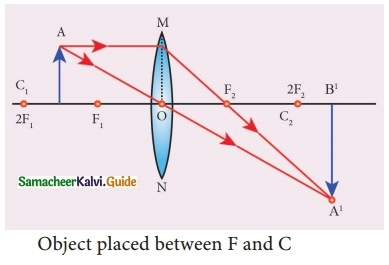Question 4.
Define dispersion of light.
When a beam of white light or composite light is refracted through any transparent media such as glass or water, it is split into its component colours. This phenomenon is called as ‘dispersion of light’.

Question 5.
State Rayleigh’s law of scattering.
Rayleigh’s scattering law states that, “The amount of scattering of light is inversely proportional to the fourth power of its wavelength”.
Amount of scattering ‘S’ ∝$$\frac{1}{λ^4}$$

Question 6.
Differentiate convex lens and concave lens.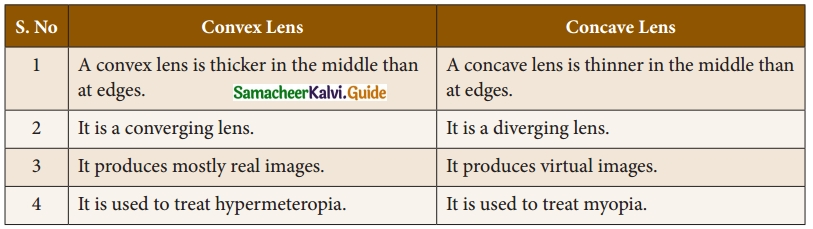Question 7.
What is the power of accommodation of the eye?

• The ability of the eye lens to focus nearby as well as the distant objects is called the power of accommodation of the eye.
• This is achieved by changing the focal length of the eye lens with the help of ciliary muscles.Question 8.
What are the causes of ‘Myopia’?

1. The lengthening of eye ball.
2. The focal length of eye lens is reduced.
3. The distance between eye lens and retina increases.
4. The far point will not be at infinity.
5. The far point comes closer.

Question 9.
Why does the sky appear blue in colour?
When sunlight passes through the atmosphere, the blue colour (shorter wavelength) is scattered to a greater extent than the red colour (longer wavelength). This scattering causes the sky to appear blue in colour.

Question 10.
Why are traffic signals red in colour?

• Red light has the highest wavelength.
• It is scattered by atmospheric particles.
• So red light is able to travel the longest distance through a fog, rain etc.

VII. Give the answer in detail.

Question 1.
List any five properties of light?

• Light is a form of energy.
• Light always travels along a straight line.
• Light does not need any medium for its propagation. It can even travel through a vacuum.
• The speed of light in vacuum or air is, c = 3 × 108 ms-1
• Since light is in the form of waves, it is characterized by a wavelength (λ) and a frequency (v), which are related by the following equation: c = vλ (c = velocity of light).
• Different coloured light has a different wavelength and frequency.

Question 2.
Explain the rules for obtaining images formed by a convex lens with the help of ray diagram.
Rule-1: When a ray of light strikes the convex or concave lens obliquely at its optical centre, it continues to follow its path without any deviation.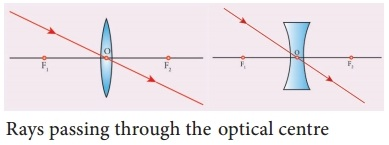Rule-2: When rays parallel to the principal axis strikes a convex or concave lens, the refracted rays are converged to (convex lens) or appear to diverge from (concave lens) the principal focus.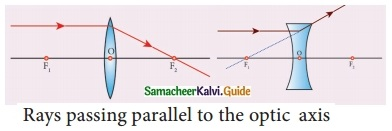Rule-3: When a ray passing through (convex lens) or directed towards (concave lens) the principal focus strikes a convex or concave lens, the refracted ray will be parallel to the principal axis.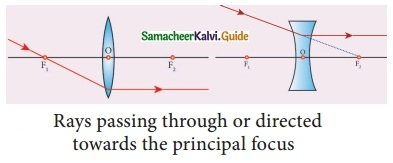Question 3.
Differentiate the eye defects: Myopia and Hypermetropia.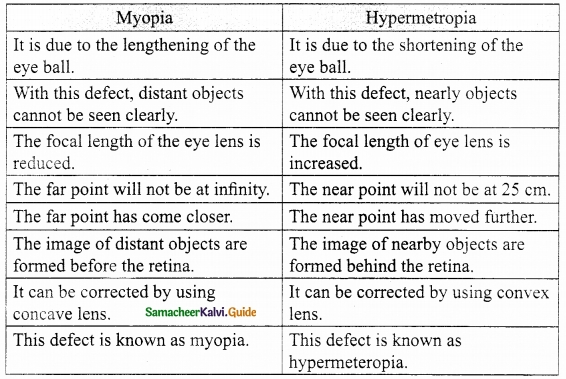Question 4.
Explain the construction and working of a ‘Compound Microscope’.
Construction : A compound microscope consists of two convex lenses. The lens with the shorter focal length is placed near the object, and is called as ‘objective lens’ or ‘objective piece’. The lens with larger focal length and larger aperture placed near the observer’s eye is called as ‘eye lens’ or ‘eye piece’. Both the lenses are fixed in a narrow tube with adjustable provision.

Working : The object (AB) is placed at a distance slightly greater than the focal length of objective lens (u > F0). A real, inverted and magnified image (A’B’) is formed at the other side of the objective lens. This image behaves as the object for the eye lens. The position of the eye lens is adjusted in such a way, that the image (B’B’) falls within the principal focus of the eye piece. This eye piece forms a virtual, enlarged and erect image (A”B”) on the same side of the object.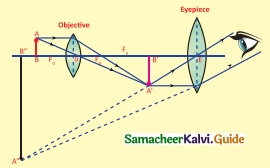Compound microscope has 50 to 200 times more magnification power than simple microscope.

VIII. Numerical Problems.

Question 1.
An object is placed at a distance 20 cm from a convex lens of focal length 10 cm. Find the image distance and nature of the image.
Distance of an object u = 20 cm
Focal length of a convex lens f = 10 cm
Let the image distance be v
We know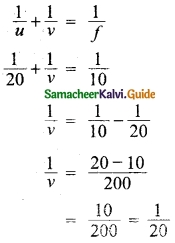v = 20 cm
Magnification m = $$\frac{v}{u}$$ = $$\frac{20}{20}$$ = 1
Hence a real image of same size is formed at 20 cm.
Image distance = 20 cm

Question 2.
An object of height 3 cm is placed at 10 cm from a concave lens of focal length 15 cm. Find the size of the image.
Object distance u = 10 cm
Focal length of a concave lens f= -15 cm
Let v be the image distance,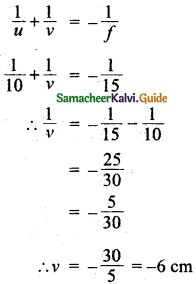Distance of image v = 6 cm
Magnification m = $$\frac{v}{u}$$ = $$\frac{6}{10}$$ = 0.6
And Magnification m = $$\frac{h’}{h}$$
Where h’ – height of image
h – height of object
0.6 = $$\frac{h’}{3}$$
∴ h’ = 3 × 0.6 = 1.8 cm
∴ Height of image = 1.8 cmIX. Higher order thinking (HOT) questions.

Question 1.
While doing an experiment for the determination of focal length of a convex lens, Raja Suddenly dropped the lens. It got broken into two halves along the axis. If he continues his experiment with the same lens,
(a) can he get the image?
(b) Is there any change in the focal length?
(a) He can get the image.
(b) The focal length of the lens will be doubled.

Question 2.
The eyes of the nocturnal birds like owl are having a large cornea and a large pupil. How does it help them?

• The large pupil opens wider and allows the maximum amount of light to enter the eye in the dark.
• Their lens is large and situated near the retina. This also allows a lot of light to register on the retina. The retina contains 2 types of light-sensing cells rods and cones.
• Cones are responsible for the coloured vision and require bright, focused light.
• Rods are extremely sensitive to light and have a photosensitive pigment called rhodopsin which plays a vital role in night vision.

Question 1.
The scattering of sun light by the atoms or molecules of the gases in the Earth’s atmosphere is known as:
(a) Mie scattering
(b) Tyndall scattering
(c) Rayleigh scattering
(d) Raman scattering
(c) Rayleigh scattering

Question 2.
Mie scattering is responsible for the _____ appearance of the clouds.
(a) red
(b) blue
(c) colourless
(d) white.
(d) white

Question 3.
In an inelastic scattering the energy of the incident beam of light is ……….. that of scattering beam.
(a) greater than
(b) less than
(c) equal to
(d) different from
(d) different from

Question 4.
As per Rayleigh’s scattering law, amount of scattering is:
(a) directly proportioanl to fourth power of wavelength
(b) inversely proportioanl to fourth power of wavelength
(c) inversely proportioanl to square of wavelength
(d) directly proportional to square of wavelength
(b) inversely proportioanl to fourth power of wavelengthQuestion 5.
The refractive index of a medium is dependent on the _____ of the light.
(a) wavelength
(b) strength
(c) density
(d) refraction.
(a) wavelength

Question 6.
The scattering of light by colloidal particles in the colloidal solution is called:
(a) Raman scattering
(b) Tyndall scattering
(c) Mie scattering
(d) Elastic scattering
(b) Tyndall scattering

Question 7.
A piece of transparent material bounded by curved surfaces is called:
(a) mirror
(b) prism
(c) slab
(d) lens
(d) lens

Question 8.
If the energy of the incident and the scattered beam of light are not the same, then it is called as _____.
(a) Elastic
(b) Raman
(c) Inelastic
(d) Mie.
(c) Inelastic

Question 9.
A convex lens does not produce:
(a) real magnified image
(b) virtual magnified image
(c) virtual diminished image
(d) real diminished image
(c) virtual diminished image

Question 10.
A lens which is thicker in the middle than at the edges is known as:
(a) concave lens
(b) convex lens
(c) bifocal lens
(d) cylindrical lens
(b) convex lens

Question 11.
The object is always placed on the _____ side of the lens.
(a) left
(b) right
(c) top
(d) bottom.
(a) left

Question 12.
The parallel rays from the outer edge are deviated towards the middle in a:
(a) convex mirror
(b) concave lens
(c) concave mirror
(d) convex lens
(d) convex lens

Question 13.
The light rays passing through the optic centre will:
(a) diverged
(b) scattered
(c) converged
(d) emerge undeviated
(d) emerge undeviated

Question 14.
All the distances are measured from the ______ of the lense.
(a) centre of curvature
(b) optical centre
(c) principal focus
(d) infinity.
(b) optical centre

Question 15.
A ray passing through the principal focus and incident on the lens will:
(a) converge
(b) diverge
(c) emerge parallel to the principal axis
(d) not emerge out
(c) emerge parallel to the principal axisQuestion 16.
When the object is placed at infinity from the convex lens, the image is formed at:
(a) F
(b) C
(c) infinity
(d) between F and 2F
(a) F

Question 17.
The human eye is ____ in nature.
(a) convex
(b) concave
(c) transparent glass
(d) Plano – concave.
(a) convex

Question 18.
The image formed by a concave lens is:
(a) virtual
(b) diminished
(c) virtual and diminished
(d) virtual and enlarged
(c) virtual and diminished

Question 19.
To get a real image using convex lens, the object must be placed at:
(a) infinity
(b) principal focus
(c) beyond principal focus and infinity
(d) both (b) and (c)
(d) both (b) and (c)

Question 20.
_____ is the centre part of the iris.
(a) cornea
(b) retina
(c) pupil
(d) eye lens.
(c) pupil

Question 21.
For a convex lens the point at which the parallel rays converge is called of the lens.
(a) pole
(b) centre of curvature
(c) principal focus
(d) none
(c) principal focus

Question 22.
A real image formed by a convex lens is always:
(a) erect
(b) magnified
(c) inverted
(d) diminished
(c) inverted

Question 23.
The law of distances is given by: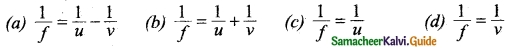(b) $$\frac{1}{f}$$ = $$\frac{1}{u}$$ + $$\frac{1}{v}$$

Question 24.
The unit of focal length is:
(a) dioptre
(b) metre
(c) ohm
(d) ampere
(b) metre

Question 25.
The sign of focal length of a convex lens is ………. sign.
(a) negative
(b) positive
(c) negative or positive
(d) none of the above
(b) positiveQuestion 26.
The focal length of concave lens has ……… sign.
(a) positive
(b) negative
(c) positive or negative
(d) none of the above
(b) negative

Question 27.
The magnification in terms of object distance u and image distance v is m :
(a) $$\frac{u}{v}$$
(b) u + v
(c) $$\frac{v}{u}$$
(d) uv
(c) $$\frac{v}{u}$$

Question 28.
In terms of object distance u and focal length/, magnification is given by m =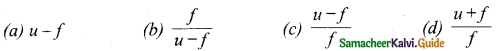(b) $$\frac{f}{u-f}$$

Question 29.
The magnification in terms of v and/:
(a) f
(b) v – f
(c) $$\frac{f}{v-f}$$
(d) $$\frac{v-f}{f}$$
(d) $$\frac{v-f}{f}$$

Question 30.
The unit of power is:
(a) m
(b) ohm
(C) dioptre
(d) ampere
(C) dioptre

Question 31.
If the focal length of a convex lens is 1 m then its power is:
(a) 1 dioptre
(b) 0.1 dioptre
(c) 10 dioptre
(d) 0.01 dioptre
(a) 1 dioptre

Question 32.
In a simple microscope, the magnification can be increased by:
(a) lens of long focal length
(b) lens
(c) lens of short focal length
(d) lens of infinite focal length
(c) lens of short focal length

Question 33.
Convex lenses are used:
(a) as camera lenses
(b) as magnifying lenses
(c) to correct hypermetropia
(d) all the above
(d) all the above

Question 34.
Which lens is used in wide angle spyhole in doors?
(a) convex lens
(b) concave lens
(c) cylindrical lens
(d) parabolic lens
(b) concave lens

Question 35.
The mathematical form of lens maker’s formula is: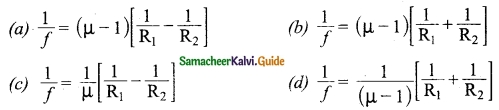(a)Question 36.
If f is the focal length of the lens then its power is given by:
(a) P = $$\frac{2}{f}$$
(b) p = $$\frac{1}{f}$$
(c) p = f
(d) p = f1
(b) p = $$\frac{1}{f}$$

Question 37.
Which part of the human eye changes the focal length of the eye lens?
(a) pupil
(b) retina
(c) ciliary muscles
(d) cornea
(c) ciliary muscles

Question 38.
On which part of human eye, image is formed?
(a) cornea
(b) iris
(c) retina
(d) pupil
(c) retina

Question 39.
For normal human eye the value of near point is:
(a) 25 cm
(b) 25 m
(c) 2.5 m
(d) 25 mm
(a) 25 cm

Question 40.
In hypermeteropia, the focal length of the eye lens is:
(a) decreased
(b) remains the same
(c) increased
(d) none of the above
(c) increased

Question 41.
Presbyopia can be corrected by using:
(a) convex lens
(b) bifocal lens
(c) concave lens
(d) cylindrical lens
(b) bifocal lens

Question 42.
Astigmatism can be corrected by using:
(a) bifocal lens
(b) cylindrical lens
(c) convex lens
(d) concave lens
(b) cylindrical lens

Question 43.
The magnifying power of compound microscope is:
(a) 10
(b) 20
(c) 50
(d) 50 to 200
(d) 50 to 200

Question 44.
The accuracy of travelling microscope is of the order of:
(a) 0.01 cm
(b) 0.01 mm
(c) 0.1 mm
(d) 0.1 cm
(b) 0.01 mmII. Fill in the blanks.

1. The velocity of light in vacuum is ……….
2. If v is the frequency and λ is the wavelength then velocity of the wave is c = ……….
3. Among colours of visible light ……… colour has the highest wavelength.
4. According to Snell’s law refractive index, µ2 = ……….
5. In a medium having high value of refractive index then speed of light in that medium is ……….
6. Angle of refraction is the smallest for ……… and the highest for ……….
7. The refractive index depends on ………. of light.
8. Colours having shorter wavelength scattered more than longer wavelength colours according to ……….. law.
9. After passing through a convex lens ……….. rays ………. at the principal focus.
10. For a convex lens, as the object distance increases, the image distance ……….
11. A ray passing through the optic centre of a lens emerges …………
12. ……… is due to irregular curvature of the surface of the eye lens.
13. When a parallel beam of light passes through a convex lens, the rays from the outer edges are …………
14. A ray parallel to the principal axis of a convex lens after refraction passes through …………
15. When the object is placed between ………….. and ………… of a convex lens a virtual image will be formed.
16. For a convex lens, as the object approaches the lens the image becomes …………
17. In a phographic camera ………… lens is used.
18. The shorter the focal length, the ………. is the magnification.
19. The nature of the image formed by a simple microscope is ……….., ………… and …………
20. Real images are formed by a ……….. lens.
21. Concave lens produces ………… images.
22. The value of power of a lens having focal length one metre is ………..
23. For a normal eye the value of far point is …………
24. …………. is known as short sightedness.
25. Hyper metropia is known as ………..
26. The mathematical form of focal length of a concave lens used to correct myopia is f = ……….
27. ……….. lenses are used to correct astigmatism.
28. For a normal eye, the value of least distance of distinct vision is ………..
29. The objective of the compound microscope has …………. focal length.
30. The focal length of ………. is greater in a compound microscope.
31. ……… is an optical instrument to see the distant objects.
32. A terrestrial telescope produces ……… image.
33. Elaborate view of galaxies and planets is obtained by ………
1. 3 × 108 m/s
2. vλ
3. red
4. $$\frac{sin i}{sin r}$$
5. low
6. red, violet
7. wavelength
8. Rayleigh scattering
9. parallel, converge
10. will decrease
11. undeviated
12. Astigmatism
13. deviated towards the centre of the lens
14. the principal focus
15. principal focus, optical centre
16. bigger
17. biconvex
18. greater
19. virtual, erect, magnified
20. convex
21. virtual
22. One dioptre
23. infinity
24. Myopia
25. long sightedness
26. xy/x – y
27. Cylindrical
28. 25 cm
29. shorter
30. eye piece
31. Telescope
32. an erect
33. TelescopeIII. True or False. If false correct it.

1. Light does not travel along a straight line.
2. All coloured light has same wavelength.
3. In refraction incident ray, refracted ray and normal lie in the same plane.
4. Velocity of light is greater in rarer medium is greater than that in denser medium.
5. For red colour angle of refraction is the least.
6. The refractive index of a medium ¡s independent of wavelength.
7. Tyndall scattering, is the scattering of light by colloids.
8. According to Rayleigh’s scattering law, red colour is scattered to a greater extent than blue colour.
9. Mie scattering takes place when the diameter is larger than the wavelength of the incident light.
10. The lines in Raman scattering having frequencies lower than the incident frequency are called Antistoke’s lines.
11. In front of a convex lens when the object is placed at infinity the formed image is smaller than that of the object.
12. When an object is placed at finite distance from the concave lens a virtual image is formed between optical centre and focus of the concave lens.
13. Pupil of human eye bends the incident light on to the lens.
14. For a normal human eye, the value of far point is 25 cm.
15. Astigmatism is corrected by cylindrical lenses.
1. False – Light always travels along a straight lines.
2. False – Different coloured light has different wavelength.
3. True
4. True
5. True
6. False – The refractive index of a medium depends on wavelength.
7. True
8. False – According to Rayleigh’s scattering law, blue colour is scattered to a greater extent than red colour.
9. True
10. False – The lines in Raman scattering having frequencies higher than the incident frequency are called Antistoke’s lines.
11. True
12. True
13. False – Cornea of human eye bends the incident light on to the lens.
14. False – For a normal human eye, the value of near point is 25 cm.
15. True

IV. Match the following.

Question 1.
Match the column I with column II.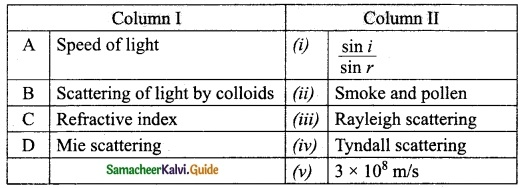A. (v)
B. (iv)
C. (i)
D. (ii)

Question 2.
Position of the object placed infront of a convex lens are given in Column
I. Match them with the natures of the images formed by the convex lens given in column II.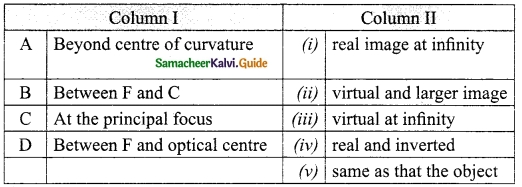A. (v)
B. (iv)
C. (i)
D. (ii)Question 3.
Match the following: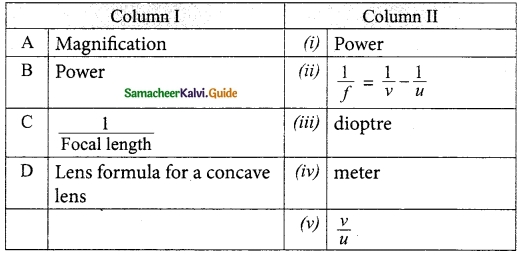A. (v)
B. (iv)
C. (i)
D. (ii)

Question 4.
Match the Column I with Column II.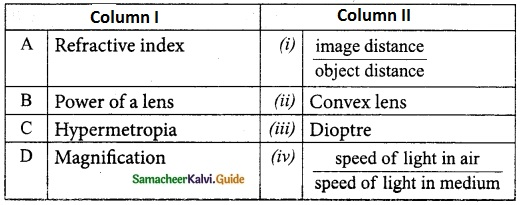A. (iv)
B. (iii)
C. (ii)
D. (i)

Question 5.
Match the Column I with Column II.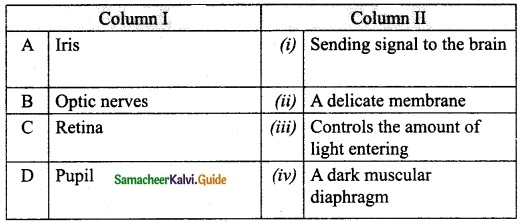A. (iv)
B. (i)
C. (ii)
D. (iii)

Question 6.
Match the column I with column II.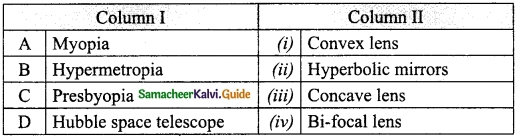A. (iii)
B. (i)
C. (iv)
D. (ii)

Question 7.
Match the Column I with Column II.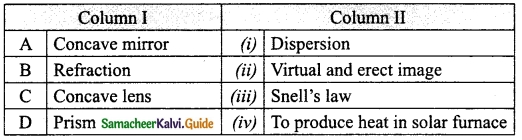A. (iv)
B. (iii)
C. (ii)
D. (i)

V. Assertion and Reasoning type.

Question 1.
Assertion : The sun looks bigger in size at sunrise and sunset than during day.
Reason : In detraction light rays bend around the edges of the obstacle.
(a) If both Assertion and Reason are true and Reason is the correct explanation of Assertion.
(b) If both Assertion and Reason are true but Reason is not the correct explanation of Assertion.
(c) If Assertion is true but Reason is false.
(d) If Assertion is false but Reason is true.
(b) If both Assertion and Reason are true but Reason is not the correct explanation of Assertion.

Question 2.
Assertion: Colours can be scan in thin layers of oil on the surface water. Reason: White light is composed of several colours.
(a) If both Assertion and Reason are true and Reason is the correct explanation of Assertion.
(b) If both Assertion and Reason are true but Reason is not the correct explanation of Assertion.
(c) If Assertion is true but Reason is false.
(d) If Assertion is false but Reason is true.
(b) If both Assertion and Reason are true but Reason is not the correct explanation of Assertion.

Question 3.
Assertion: Raman spectrum of a liquids contains lines whose frequencies are not equal to that of incident radiation.
Reason: If a photon strikes an atom in a liquid that is in existed state photon losses energy.
(a) If both Assertion and Reason are true and Reason is the correct explanation of Assertion.
(b) If both Assertion and Reason are true but Reason is not the correct explanation of Assertion.
(c) If Assertion is true but Reason is false.
(d) If Assertion is false but Reason is true.
(b) If both Assertion and Reason are true but Reason is not the correct explanation of Assertion.Question 4.
Assertion: The refractive index of a prism depends only on the material of the prism.
Reason: The refractive index of a prism depends upon the refracting angle and angel of minimum deviation.
(a) If both Assertion and Reason are true and Reason is the correct explanation of Assertion.
(b) If both Assertion and Reason are true but Reason is not the correct explanation of Assertion.
(c) If Assertion is true but Reason is false.
(d) If Assertion is false but Reason is true.
(c) If Assertion is true but Reason is false.

Question 5.
Assertion: A single lens produces a coloured image of an object illuminated by white light.
Reason: The refractive index of material of lens is different for different wavelength of light.
(a) If both Assertion and Reason are true and Reason is the correct explanation of Assertion.
(b) If both Assertion and Reason are true but Reason is not the correct explanation of Assertion.
(c) If Assertion is true but Reason is false.
(d) If Assertion is false but Reason is true.
(a) If both Assertion and Reason are true and Reason is the correct explanation of Assertion.

Question 6.
Assertion: If a convex lens is placed in water, its convergence power decrease.
Reason: Focal length of lens is independent of refractive index of the medium.
(a) If both Assertion and Reason are true and Reason is the correct explanation of Assertion.
(b) If both Assertion and Reason are true but Reason is not the correct explanation of Assertion.
(c) If Assertion is true but Reason is false.
(d) If Assertion is false but Reason is true.
(c) If Assertion is true but Reason is false.

Question 7.
Assertion: Light waves travel in straight lines.
Reason: Rectilinear propagation of light confirm the above mentioned properly.
(a) If both Assertion and Reason are true and Reason is the correct explanation of Assertion.
(b) If both Assertion and Reason are true but Reason is not the correct explanation of Assertion.
(c) If Assertion is true but Reason is false.
(d) If Assertion is false but Reason is true.
(a) If both Assertion and Reason are true and Reason is the correct explanation of Assertion.

Question 8.
Assertion: Raman scattering the scattering of monochromatic light by atoms and molecule of a liquid.
Reason: The wavelength of Raman lines is same.
(a) If both Assertion and Reason are true and Reason is the correct explanation of Assertion.
(b) If both Assertion and Reason are true but Reason is not the correct explanation of Assertion.
(c) If Assertion is true but Reason is false.
(d) If Assertion is false but Reason is true.
(b) If both Assertion and Reason are true but Reason is not the correct explanation of Assertion.

Question 9.
Assertion: Power of a lens is the reciprocal of its focal length.
Reason: The unit of power is one dioptre when the unit of focal length is one metre.
(a) If both Assertion and Reason are true and Reason is the correct explanation of Assertion.
(b) If both Assertion and Reason are true but Reason is not the correct explanation of Assertion.
(c) If Assertion is true but Reason is false.
(d) If Assertion is false but Reason is true.
(a) If both Assertion and Reason are true and Reason is the correct explanation of Assertion.

Question 10.
Assertion: Presbyopia is due to ageing of human beings.
Reason: For those persons, ciliary muscles of the eye become weak.
(a) If both Assertion and Reason are true and Reason is the correct explanation of Assertion.
(b) If both Assertion and Reason are true but Reason is not the correct explanation of Assertion.
(c) If Assertion is true but Reason is false.
(d) If Assertion is false but Reason is true.
(a) If both Assertion and Reason are true and Reason is the correct explanation of Assertion.Question 1.
What is meant by refraction?
When a ray of light travels from one transparent medium into another obliquely, the path of the light undergoes deviation. This deviation of ray of light is called refraction.

Question 2.
State laws of refraction.
First law of refraction: The incident ray, the refracted ray of light and the normal to the refracting surface all lie in the same plane.

Second law of refraction: The ratio of the sine of the angle of incidence and sine of the angle of refraction is equal to the ratio of refractive indices of the two media. This law is also known as Snell’s law.
$$\frac{sin i}{sin r}$$ = $$\frac{µ_2}{µ_1}$$

Question 3.
Define refractive index of a medium.
The ratio of speed of light in vacuum to the speed of light in a medium is defined as refractive index ‘p’ of that medium.

Question 4.
What is meant by monochromatic source?
If a source of light produces a light of single colour, it is known as a monochromatic source.

Question 5.
When white light is refracted by a transparent medium what will you get? Why?

1. When white light is refracted by a transparent medium, a spectrum is obtained.
2. This is because, different coloured lights are bent through different angles.

Question 6.
What is scattering of light?
When sunlight enters the Earth’s atmosphere, the atoms and molecules of different gases present in the atmosphere refract the light in all possible directions. This is called as ‘Scattering of light’.Question 7.
State the types of scattering.

1. Elastic scattering
2. Inelastic scattering

Question 8.
What is elastic scattering?
If the energy of the incident beam of light and the scattered beam of light are same, then it is called as ‘elastic scattering’.

Question 9.
What is inelastic scattering?
If the energy of the incident beam of light and the scattered beam of light are not same, then it is called as ‘inelastic scattering’.

Question 10.
How are different types of scattering formed? Mention the types of scattering.
The nature and size of the scatterer results in different types of scattering. They are

1. Rayleigh scattering
2. Mie scattering
3. Tyndall scattering
4. Raman scattering

Question 11.
What is Rayleigh scattering?
The scattering of sunlight by the atoms or molecules of the gases in the earth’s atmosphere is known as Rayleigh scattering.

Question 12.
Why the colour of the Sun is red at sunrise and sunset?
At sunrise and sunset, the light rays from the Sun have to travel a larger distance in the atmosphere than at noon. Hence, most of the blue lights are scattered away and only the red light which gets least scattered reaches us. Therefore, the colour of the Sun is red at sunrise and sunset.

Question 13.
When does Mie scattering take place?
Mie scattering takes place when the diameter of the scatterer is similar to or larger than the wavelength of the incident light.

Question 14.
What are the causes of Mie scattering?
Mie scattering is caused by pollen, dust, smoke, water droplets, and other particles in the lower portion of the atmosphere.

Question 15.
Why the clouds have white appearance?
Mie scattering is responsible for the white appearance of the clouds. When white light falls on the water drop, all the colours are equally scattered which together form the white light.

Question 16.
What is Tyndall Scattering?
The scattering of light rays by the colloidal particles in the colloidal solution is called Tyndall Scattering or Tyndall Effect.

Question 17.
What is meant by colloid? State few examples.
Colloid is a microscopically small substance that is equally dispersed throughout another material. Eg: Milk, Ice cream, muddy water, smoke.Question 18.
What is meant by Raman Scattering?
When a parallel beam of monochromatic (single coloured) light passes through a gas or liquid or transparent solid, a part of light rays are scattered.

Question 19.
Define Raman Scattering.
Raman Scattering is defined as “The interaction of light ray with the particles of pure liquids or transparent solids, which leads to a change in wavelength or frequency.”

Question 20.
What is Rayleigh line?
The spectral lines having frequency equal to the incident ray frequency is called ‘Rayleigh line’.

Question 21.
What are Raman lines?
The spectral lines which are having frequencies other than the incident ray frequency are called ‘Raman lines’.

Question 22.
What are stokes lines and Antistokes lines?
The lines having frequencies lower than the incident frequency is called stokes lines and the lines having frequencies higher than the incident frequency are called Antistokes lines.

Question 23.
What is a lens?
A lens is an optically transparent medium bounded by two spherical refracting surfaces or one plane and one spherical surface.

Question 24.
How is lens classified?
Lens is basically classified into two types. They are:

1. Convex Lens
2. Concave Lens.

Question 25.
What is biconvex lens?
Convex or bi-convex lens: It is a lens bounded by two spherical surfaces such that it is thicker at the centre than at the edges. A beam of light passing through it, is converged to a point. So, a convex lens is also called as converging lens.

Question 26.
What is meant by biconcave lens?
Concave or bi-concave Lens: It is a lens bounded by two spherical surfaces such that it is thinner at the centre than at the edges. A parallel beam of light passing through it, is diverged or spread out. So, a concave lens is also called as diverging lens.

Question 27.
What are
(i) Plano-convex lens?
(ii) Plano-concave lens?
(i) If one of the faces of a bi-convex lens is plane, it is known as a plano-convex lens.
(ii) If one of the faces of a bi-concave lens is plane, it is known as a plano-concave lens.

Question 28.
State the applications of convex lenses.

1. Convex lenses are used as camera lenses.
2. They are used as magnifying lenses.
3. They are used in making microscope, telescope and slide projectors.
4. They are used to correct the defect of vision called hypermetropia.Question 29.
Draw diagrams of different types converging lenses.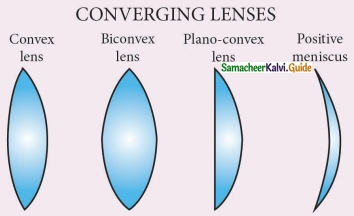Question 30.
Represent different types of lenses by diagram.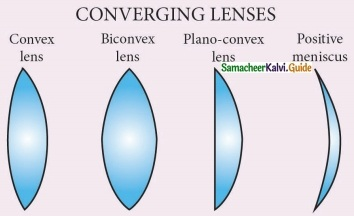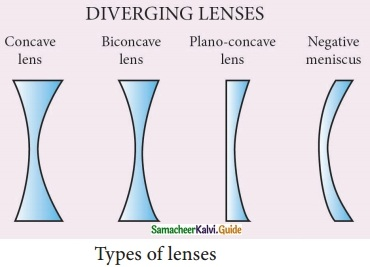Question 31.
What is the nature of the image formed by an object is placed behind the centre of curvature of a convex leas. Draw a ray diagram.
When an object is placed behind the center of curvature (beyond C), a real and inverted image is formed between the center of curvature and the principal focus. The size of the image is the same as that of the object.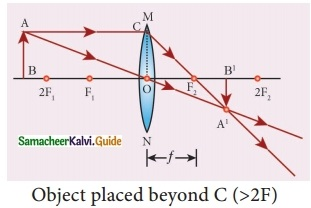Question 32.
Draw a ray diagram to indicate the nature of the image formed when an object is placed in between the centre of curvature and principal focus of a convex lens.
When an object is placed in between the center of curvature and principal focus, a real and inverted image is formed behind the center of curvature. The size of the image is bigger than that of the object.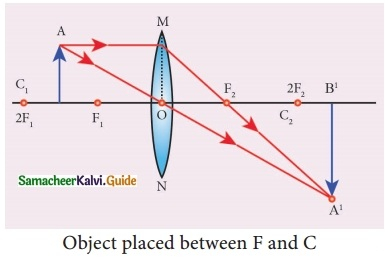Question 33.
Draw a ray diagram for the formation of image (by the concave lens) when object is at infinity.
When an object is placed at infinity, a virtual image is formed at the focus. The size of the image is much smaller than that of the object.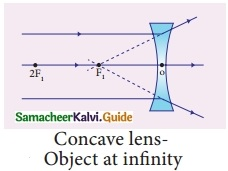Question 34.
What are the applications of concave lens?

1. Concave lenses are used as eye lens of‘Galilean Telescope’.
2. They are used in wide angle spy hole in doors.
3. They are used to correct the defect of vision called ‘myopia’.Question 35.
What do you know about lens formula?
The lens formula gives the relationship among distance of the object (u), distance of the image (v) and the focal length (f) of the lens. It is expressed as
$$\frac{1}{f}$$ = $$\frac{1}{v}$$ – $$\frac{1}{u}$$

Question 36.
Define magnification.
It is defined as the ratio of the height of the image to the height of an object. Magnification is denoted by the letter ‘m’. If height of the object is h and height of the image is h’, the magnification produced by lens is,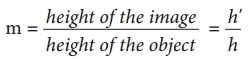Question 37.
What is lens formula?
The lens formula relates the focal length of a lens with the distance of object and image.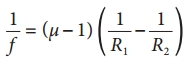where µ is the refractive index of the material of the lens; R1 and R2 are the radii of curvature of the two faces of the lens; f is the focal length of the lens.

Question 38.
What is meant by power of lens?
The ability of a lens to converge (convex lens) or diverge (concave lens) light rays is called as its power.

Question 39.
Define power of a lens. State its unit.
Power of a lens is numerically defined as the reciprocal of its focal length.
P = $$\frac{1}{f}$$
The SI unit of power of a lens is dioptre.

Question 40.
What is meant by dioptre.
Dioptre is the power of a lens, whose focal length is 1 metre.
1 Dioptre = 1 m-1.

Question 41.
Differentiate convex lens from concave lens.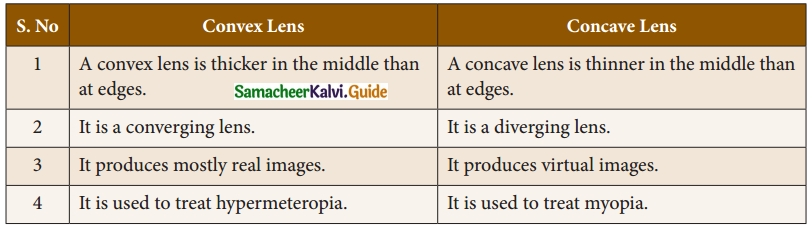Question 42.
What are
(i) Pupil &
(ii) Retina?
(i) Pupil: It is the centre part of the Iris. It is the pathway for the light to retina.
(ii) Retina: This is the back surface of the eye. It is the most sensitive part of human eye, on which real and inverted image of objects is formed.

Question 43.
What is persistence of vision?
If the time interval between two consecutive light pulses is less than 0.1 second, human eye cannot distinguish them separately. It is called persistence of vision.Question 44.
What is least distance of distinct vision?
The minimum distance required to see the objects distinctly without strain is called least distance of distinct vision. It is called as near point of eye. It is 25 cm for normal human eye.

Question 45.
What is far point?
The maximum distance up to which the eye can see objects clearly is called as far point of the eye.

Question 46.
What is Presbyopia?
Due to ageing, ciliary muscles become weak and the eye-lens become rigid (inflexible) and so the eye loses its power of accommodation. Because of this, an aged person cannot see the nearby objects clearly. So, it is also called as ‘old age hypermetropia’.

Question 47.
What is meant by astigmatism?
In this defect, eye cannot see parallel and horizontal lines clearly. It may be inherited or acquired. It is due to the imperfect structure of eye lens because of the development of cataract on the lens, ulceration of cornea, injury to the . refracting surfaces, etc. Astigmatism can be corrected by using cylindrical lenses (Torrid lenses).

Question 48.
State the principle of microscope. How is it classified?
It works under the principle of angular magnification of lenses. It is classified as

1. Simple microscope
2. Compound microscope

Question 49.
What are the uses of simple microscope?
Simple microscopes are used

1. By watch repairers and jewellers.
2. To read small letters clearly.
3. To observe parts of flower, insects etc.
4. To observe finger prints in the field of forensic science.

Question 50.
How is telescope classified?
According to optical property, it is classified into two groups:

1. refracting telescope
2. reflecting telescope

Question 51.

1. Elaborate view of the Galaxies, Planets, stars and other heavenly bodies is possible.
2. Camera can be attached for taking photograph for the celestial objects.
3. Telescope can be viewed even with the low intensity of light.

Question 52.
What are the disadvantages of telescope?

1. Frequent maintenance is needed.
2. It is not easily portable.VII. Give the answer in Detail.

Question 1.
State the Laws of Refraction.
The incident ray, the refracted ray of light and the normal to the refracting surface all lie in the same plane.
Second law of Refraction:

1. The ratio of the sine of the angle of incidence and sine of the angle of refraction is equal to the ratio of refractive indices of the two media. This law is also known as Snell’s law.
$$\frac{\sin i}{\sin r}=\frac{\mu_{2}}{\mu_{1}}$$
2. Refractive index gives us an idea of how fast or how slow light travels in a medium. The ratio of the speed of light in a vacuum to the speed of light in a medium is defined as the refractive index ‘µ’ of that medium.
3. The speed of light in a medium is low if the refractive index of the medium is high and vice versa.
4. When light travels from a denser medium into a rarer medium, the refracted ray is bent away from the normal drawn to the interface.
5. When light travels from a rarer medium into a denser medium, the refracted ray is bent towards the normal drawn to the interface.

Question 2.
Describe Raman Scattering.
When a parallel beam of monochromatic (single coloured) light passes through a gas or liquid or transparent solid, a part of light rays are scattered.

The scattered light contains some additional frequencies (or wavelengths) other than that of incident frequency (or wavelength). This is known as Raman scattering or Raman Effect.

Raman Scattering is defined as “The interaction of light ray with the particles of pure liquids or transparent solids, which leads to a change in wavelength or frequency.”

The spectral lines having frequency equal to the incident ray frequency is called ‘Rayleigh line’ and the spectral lines which are having frequencies other than the incident ray frequency are called ‘Raman lines’. The lines having frequencies lower than the incident frequency is called stokes lines and the lines having frequencies higher than the incident frequency are called Antistokes lines.

Question 3.
With the help of ray diagram, explain the nature, size and position of the image formed by a convex lens. When object is placed at
(i) infinity
(ii) beyond C
(iii) placed at C
(iv) Placed between F and C,
(v) placed at F
(vi) placed between F and optical centre O.
(i) Object at infinity: When an object is placed at infinity, a real image is formed at the principal focus. The size of the image is much smaller than that of the object.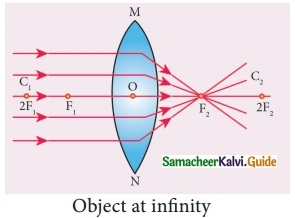(ii) Object placed beyond C (>2F): When an object is placed behind the center of curvature(beyond C), a real and inverted image is formed between the center of curvature and the principal focus. Th e size of the image is the same as that of the object.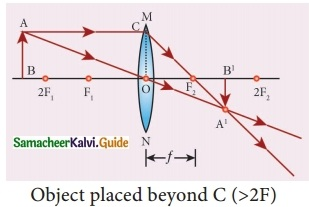(iii) Object placed at C: When an object is placed at the center of curvature, a real and inverted image is formed at the other center of curvature. The size of the image is the same as that of the object.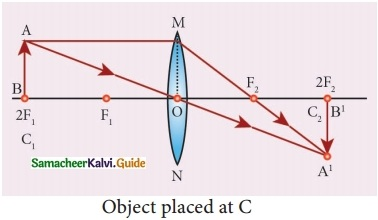(iv) Object placed between F and C: When an object is placed in between the center of curvature and principal focus, a real and inverted image is formed behind the center of curvature. The size of the image is bigger than that of the object.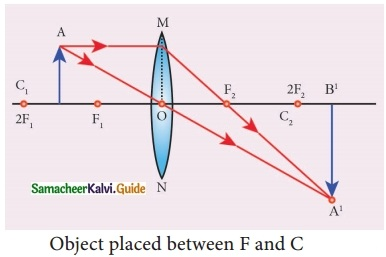(v) Object placed at the principal focus F: When an object is placed at the focus, a real image is formed at infinity. The size of the image is much larger than that of the object.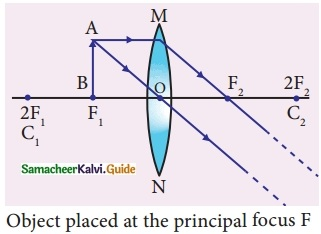(vi) Object placed between the principal focus F and optical centre O: When an object is placed in between principal focus and optical centre, a virtual image is formed. The size of the image is larger than that of the object.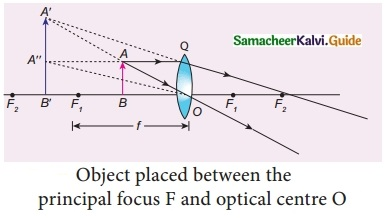Question 4.
Explain the formation of images formed by a concave lens.
Object at Infinity: When an object is placed at infinity, a virtual image is formed at the focus. The size of the image is much smaller than that of the object.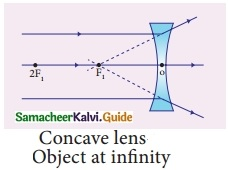Object anywhere on the principal axis at a finite distance: When an object is placed at a finite distance from the lens, a virtual image is formed between optical center and focus of the concave lens. The size of the image is smaller than that of the object.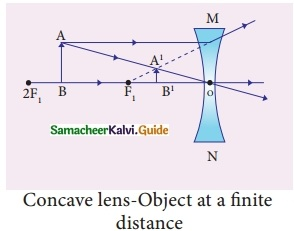But, as the distance between the object and the lens is decreased, the distance between the image and the lens also keeps decreasing. Further, the size of the image formed increases as the distance between the object and the lens is decreased.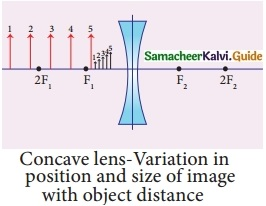Question 5.
Explain Mie Scattering.
Mie scattering:

1. Mie scattering takes place when the diameter of the Scatterer is similar to or larger than the wavelength of the incident light. It is also an elastic scattering.
2. The amount of scattering is independent of wavelength.
3. Mie scattering is caused by pollen, dust, smoke, water droplets, and other particles in the lower portion of the atmosphere.
4. Mie scattering is responsible for the white appearance of the clouds.
5. When white light falls on the water drop, all the colours are equally scattered which, together form the white light.

Question 6.
With the help of a diagram, explain the structure and working of human eye.
Structure of the eye:
The eye ball is approximately spherical in shape with a diameter of about 2.3 cm. It consists of a tough membrane called sclera, which protects the internal parts of the eye.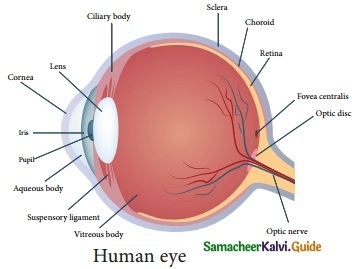Cornea : This is the thin and transparent layer on the front surface of the eyeball as shown in figure. It is the main refracting surface. When light enters through the cornea, it refracts or bends the light on to the lens.

Iris : It is the coloured part of the eye. It may be blue, brown or green in colour. Every person has a unique colour, pattern and texture. Iris controls amount of light entering into the pupil like camera aperture.

Pupil : It is the centre part of the Iris. It is the pathway for the light to retina.

Retina : This is the back surface of the eye. It is the most sensitive part of human eye, on which real and inverted image of objects is formed.

Ciliary muscles : Eye lens is fixed between the ciliary muscles. It helps to change the focal length of the eye lens according to the position of the object.

Eye Lens : It is the important part of human eye. It is convex in nature.

Working of the eye : The transparent layer cornea bends the light rays through pupil located at the centre part of the Iris. The adjusted light passes through the eye lens. Eye lens is convex in nature. So, the light rays from the objects are converged and a real and inverted image is formed on retina. Then, retina passes the received real and inverted image to the brain through optical nerves. Finally, the brain senses it as erect image.

Question 7.
Describe simple microscope.
Simple microscope: It has a convex lens of short focal length. It is held near the eye to get enlarged image of small objects.
Let an object (AB) is placed at a point within the principal focus (u < f) of the convex lens and the observer’s eye is placed just behind the lens. As per this position the convex lens produces an erect, virtual and enlarged image (A’B’), The image formed is in the same side of the object and the distance equal to the least distance of distinct vision (D) (For normal human eye D = 25 cm).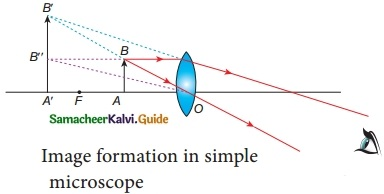Question 8.
Write short notes on
(i) Astronomical telescope
(ii) Terrestrial telescope.
(i) Astronomical Telescope: An astronomical telescope is used to view heavenly bodies like stars, planets, galaxies and satellites.

(ii) Terrestrial Telescope: The image in an astronomical telescope is inverted. So, it is not suitable for viewing objects on the surface of the Earth. Therefore, a terrestrial telescope is used. It provides an erect image. The major difference between astronomical and terrestrial telescope is erecting the final image with respect to the object.

VIII. Numerical Problems.

Question 1.
A needle of size 5 cm is placed 45 cm from a lens produced an image on a screen placed 90 cm away from the lens.
(i) Identify the types of lens.
Calculate focal length of the lens.
Height of the object h1 = 5 cm
Distance of the object u = -45 cm
Distance of the image v = 90 cm
We know that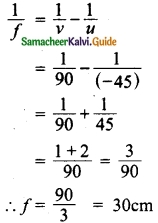Focal length of the lens = 30 cm
Since focal length is positive the lens is convex lens.

(ii) Identify the size of the image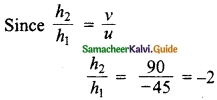∴ h2 = -10 cmQuestion 2.
A concave lens has focal length of 15 cm. At what distance should the object from the lens be placed so that it forms an image 10 cm from the lens?
v = -10 cm; f =-15 cm; u = ?
Lens formula:
$$\frac{1}{f}$$ = $$\frac{1}{v}$$ – $$\frac{1}{u}$$
Type of lens: Concave lens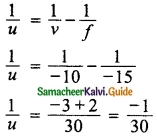u =-30 cm
Thus, the object distance is 30 cm.

Question 3.
An object is placed at a distance of 30 cm from a concave lens of focal length 15 cm. An erect and virtual image is formed at a distance of 10 cm from the lens. Calculate the magnification.
Type of lens is Cancave lens.
Formula:
Magnification m = $$\frac{v}{u}$$
Object distance u = -30 cm
Image distance v = -10 cm
m = $$\frac{-10}{-30}$$ = $$\frac{1}{3}$$ = +0.33

Question 4.
The focal length of a concave lens is 2 cm. Calculate the power of the lens.
Formula:
P = $$\frac{1}{f}$$
Type of lens is concave lens.
Focal length of concave lens,
f = -2 m power of the lens.
P = $$\frac{1}{-2 m}$$
P = -0.5 dioptre

Question 5.
A needle placed at 30 cm from the lens forms an image on a screen placed 60 cm on the other side of the lens. Identify the type of lens and determine the focal length.
u = -30 cm
v = 60 cm
u is negative because image is formed on the on the other side of the lens.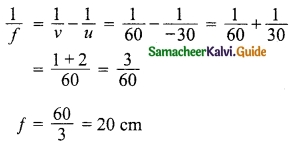It is a convex lens.

Question 6.
A 3 cm tall bulb is placed at a distance of 20 cm from a diverging lens having a focal length of 10.5 cm. Determine the distance of the image.
u = -20 cm
f = -10.5 cm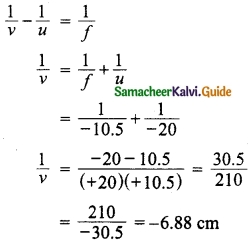The distance of the image is -6.88 cm

Question 7.
A ray from medium 1 is refracted below while passing through medium 2. Find the refractive index of the second medium with respect to medium 1.
Refractive index µ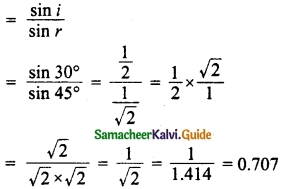Refractive index = 0.707

Question 8.
The optical prescription of a pair of spectacle is
Right eye: -3.5 D, Left eye: -4.00 D.
(i) Name the defect of the eye.
Shortsighted (Myopia)

(ii) Are these lenses thinner at the middle or at the edges?
These lenses are thinner in the middle.

(iii) Which lens has a greater focal length?
power = $$\frac{1}{focal length}$$
Right eye: power P = -3.5 D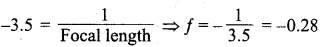Left eye: Power P = -4 D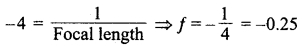Hence the lens having power of -3.5 D has greater focal length.Question 9.
The radii of curvature of two surfaces of a double convex lens are 10 cm each. Calculate its focal length and power of the lens in air and liquid. Refractive indices of glass and liquid are 1.5 and 1.8 respectively.
Radius of curvature of first surface R1 = 10 cm
Radius of curvature of second surface R2 = 10 cm
In air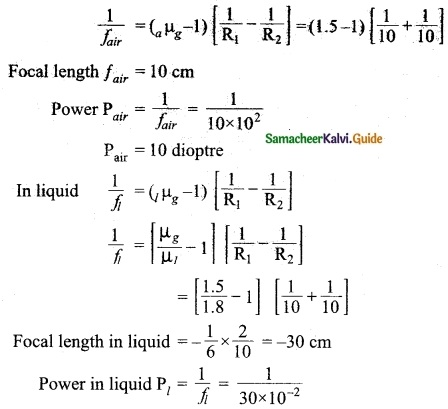pl = -3.33 d

Question 10.
An object 2 cm tall is placed 10 cm in front of a convex lens of focal length 15 cm. Find the position, size and nature of the image formed.
Focal length of a convex lens f = 15 × 10-2 m
Weight of the object ho = 2 × 10-2 m
Let weight of the image be hv
Distance of the object u = 10 × 10-2 m
Distance of the image v = 15 × 10-2 m
We know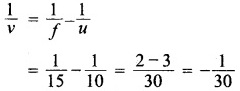∴ v = -30 × 10-2 m
Distance of the image = 30 × 10-2 m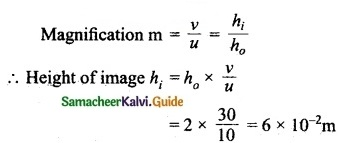Hence a virtual image 6 × 10-2 m height is formed at a distance of 30 × 10-2 m from the lens on the same side of the lens.

IX. Higher order thinking (HOT) questions.

Question 1.
Ramu passes white light through a quartz prism. For which colour refractive index is greater?
Refractive index is maximum for violet light when white light passes through a quartz prism.

Question 2.
Sita has kept a stud consists of diamond. What will she observe? Give reason.
The diamond stud appears bright because of total internal reflection.

Question 3.
Guna passes a ray light through a glass slab. Which optical phenomenon will take place? What can he observe with reference to wavelength?
When a ray of light enters a glass slab he can observe refraction of light. He observed that wavelength of light decreases.

Question 4.
A prism is placed in the minimum deviation position. Chari has passed a ray of light at an angle of 45°, then what is the value of angle of emergence? Why?
The angle of emergence = 45°.
Since, in the minimum deviation positron, angle of incidence is equal to angle of emergence.Question 5.
Mani is using a lens of power 2 dioptre. What is the focal length of the lens?
Focal length = $$\frac{1}{power}$$
F= $$\frac{1}{2}$$ = 0.5 m